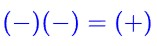# Why (-)(-)=(+)? The Question That Challenged Me When I Was in High School.When I was in high school, I had a question in mind about why multiplying two negative numbers results in a positive number. I asked my teacher about it, but was told that the question was premature for my age. Undeterred, I attempted to derive the answer on my own using the following method

Step 1:AlsoEquations (1) and (2) serve as axioms,

To proofLet,By plugging equation (1), thenBy cancelling (+) in both sides, then we obtain:

By cancelling (+) in both sides of the equation, then we obtain,Thus, the result we obtained contradicts equation (2). Then must,Then Let,By plugging equation (3),By cancelling (-) in both sides, then we obtain:Thus, the result we obtained contradicts equation (2). , then must,